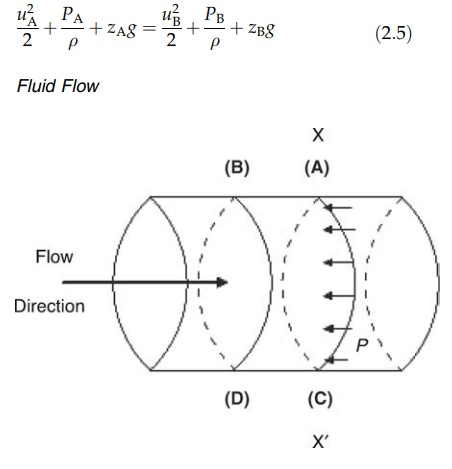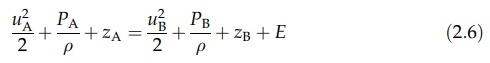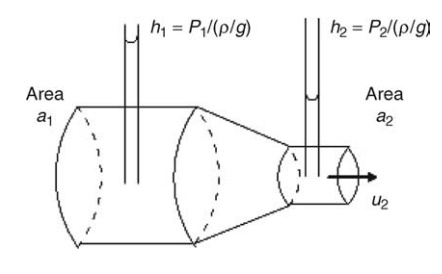# Bernoulli’s Theorem

| Home | | Pharmaceutical Technology |

## Chapter: Pharmaceutical Engineering: Fluid Flow

At any point in system through which a fluid is flowing, the total mechanical energy can be expressed in terms of the potential energy, pressure energy, and kinetic energy.

BERNOULLI’S THEOREM

At any point in system through which a fluid is flowing, the total mechanical energy can be expressed in terms of the potential energy, pressure energy, and kinetic energy. The potential energy of a body is its capacity to do work by reason of its position relative to some center of attraction. For unit mass of fluid at a height z above some reference level, potential energy = zg, where g is the acceleration due to gravity.

The pressure energy or flow energy is an energy form peculiar to the flow of fluids. The work done and the energy acquired in transferring the fluid is the product of the pressure, P, and the volume. The volume of unit mass of the fluid is the reciprocal of the density, ρ. For an incompressible fluid, the density is not dependent on the pressure, so for unit mass of fluid, pressure energy = P/ρ (Fig. 2.5).

The kinetic energy is a form of energy possessed by a body by reason of its movement. If the mass of the body is m and its velocity is u, the kinetic energy is 1/2mu2, and for unit mass of fluid, kinetic energy = u2/2.

The total mechanical energy of unit mass of fluid is, therefore,

u2/2 + P/ρ + zgThe mechanical energy at two points A and B will be the same if no energy is lost or gained by the system. Therefore, we can writeFIGURE 2.5 Pressure energy of a fluid.

This relationship neglects the frictional degradation of mechanical energy, which occurs in real systems. A fraction of the total energy is dissipated in overcoming the shear stresses induced by velocity gradients in the fluid. If the energy lost during flow between A and B is E, then equation (2.5) becomesThis is a form of Bernoulli’s theorem, restricted in application to the flow of incompressible fluids. Each term is expressed in absolute units, such as N·m/kg. The dimensions are L2T-2. In practice, each term is divided by g (LT-2) to give the dimension of length. The terms are then referred to as velocity head, pres-sure head, potential head, and friction head, the sum giving the total head of the fluid as shown in equation (2.2).The evaluation of the kinetic energy term requires consideration of the variation in velocity found in the direction normal to flow. The mean velocity, calculated by dividing the volumetric flow by the cross-sectional area of the pipe, lies between 0.5 and 0.82 times the maximum velocity found at the pipe axis. The value depends on whether flow is laminar or turbulent, terms that are described later. The mean kinetic energy, given by the term u2mean/2 differs from the true kinetic energy found by summation across the flow direction.

The former can be retained, however, if a correction factor, a, is introduced, then the

velocity head = u2mean / 2ga

where a has a value of 0.5 in laminar flow and approaches unity when flow is fully turbulent.FIGURE 2.6 Flow through a constriction.

A second modification may be made to equation (2.5) if mechanical energy is added to the system at some point by means of a pump. If the work done, in absolute units, on a unit mass of fluid is W, thenThe power required through a system at a certain rate to drive a liquid may be calculated using equation (2.8). The changes in velocity, pressure, height, and the mechanical losses due to friction are each expressed as a head of liquid. The sum of heads, ΔH, being the total head against which the pump must work, is therefore

W/g + ΔH

If the work performed and energy acquired by unit mass of fluid is ΔHg, the power required to transfer mass m in time t is given by

Power = ΔHgm/t

Since the volume flowing in unit time Q is m/ρt,

Power = QΔHgρ                          (2:9)